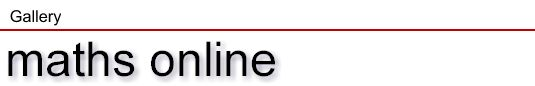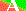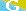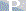The Gallery consists of interactive multimedia learning units on various issues that shall facilitate understanding. Technically, most units are Java applets, some are graphical supplements or other programs (JavaScript). For practice and (self) control, visit the collection of interactive tests. A more systematic outline of the underlying mathematical background is included in the German version of maths online. (It is one of our future plans to translate this part into English language). The Gallery is under construction since March 1998, and new learning units are added continuously. We appreciate feedback about your experience with our material.

The learning units of the Gallery

You may call the learning units directly by clicking the respective iconfor applets,for graphics or sequences of graphics, andfor other programs
A click on a hyperlink leads you to a page collecting and briefly describing all learning units of a chapter. Occasionally, remarks on additional material and further information on the web are made.

Sets

 Definition of sets

Drawing plane and coordinate system

 Cartesian coordinates Coordinate system Polar coordinates Oblique coordinates

Variables, terms, formulae, and identities

 Recognize structures 1  (use abbreviations) Recognize structures 2  (formal properties of expressions) Recognize structures 3  (numerical properties of expressions)

Equations

 Equivalence transformations Quadratic equations 1  (Proof of solution formula) Quadratic equations 2  (Three solution methods)

Vectors 1

 Introducing 3-vectors

Analytic geometry 1

 The slope of a straight line Spatial coordinates Straight lines in 3-space

Functions 1

 Function and graphGraphs of simple power functions Recognize functions 1  (simple polynomial functions) Recognize graphs 1  (functions of at most second order) Polynomial of third order (cubic polynomial)Function plotter

Trigonometric functions

 Definition of the trig functions

Trigonometry

 Triangle and Law of Sines

Functions 2

 Recognize functions 2  (functions containing negative powers) Recognize graphs 2  (functions containing negative powers) The graphs of sin, cos and tanGraphs of elementary trigonometric functionsGraphs of some exponential and logarithm functions Recognize functions 3  (sine and cosine functions) Recognize graphs 3  (sine and cosine functions)Function plotter

Limiting processes

Analytic geometry 2

 Planes in 3-space

Differentiation 1

 On the definition of the derivative Derivative puzzle 1 Derivative puzzle 2 Derivative puzzle 3 First and second derivative

Applications of differential calculus

 How to find a function's extremum

Power series

 Power series 1  (input by scroll bar) Power series 2  (numerical input)Function plotter

Analytic geometry 3

 Cycloids Logarithmic spirals

Differentiation 2

 Nowhere differentiable functions

Probability and statistics 1

 The Gauss distribution

Integration

 Intuitively understanding the integral

Mathematical structures and spaces

 Lorentz transformation

Fourier series

 Fourier series

Model-building and simulation

 Game of Life HighLife

Maths by playing

 Warp image